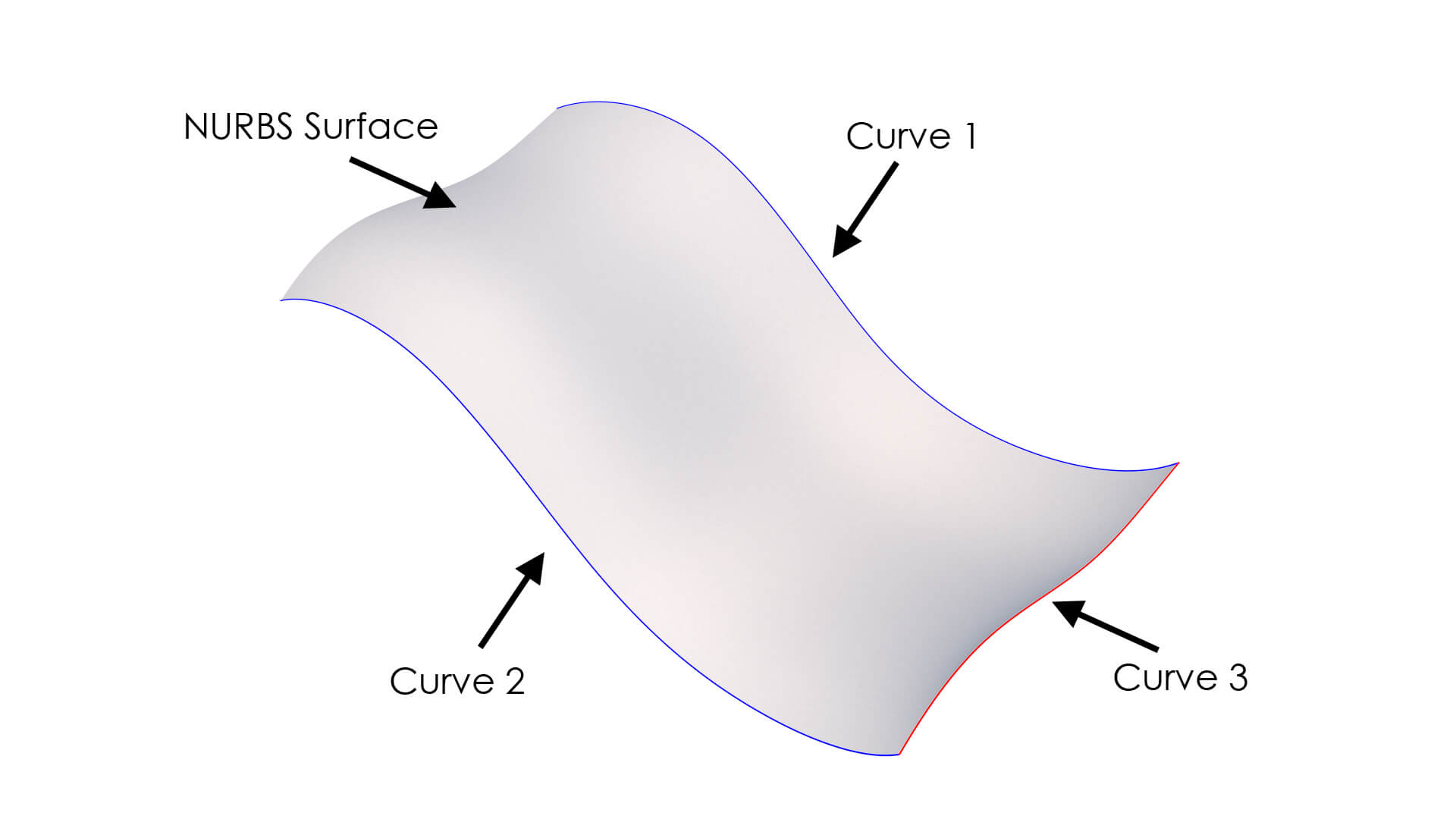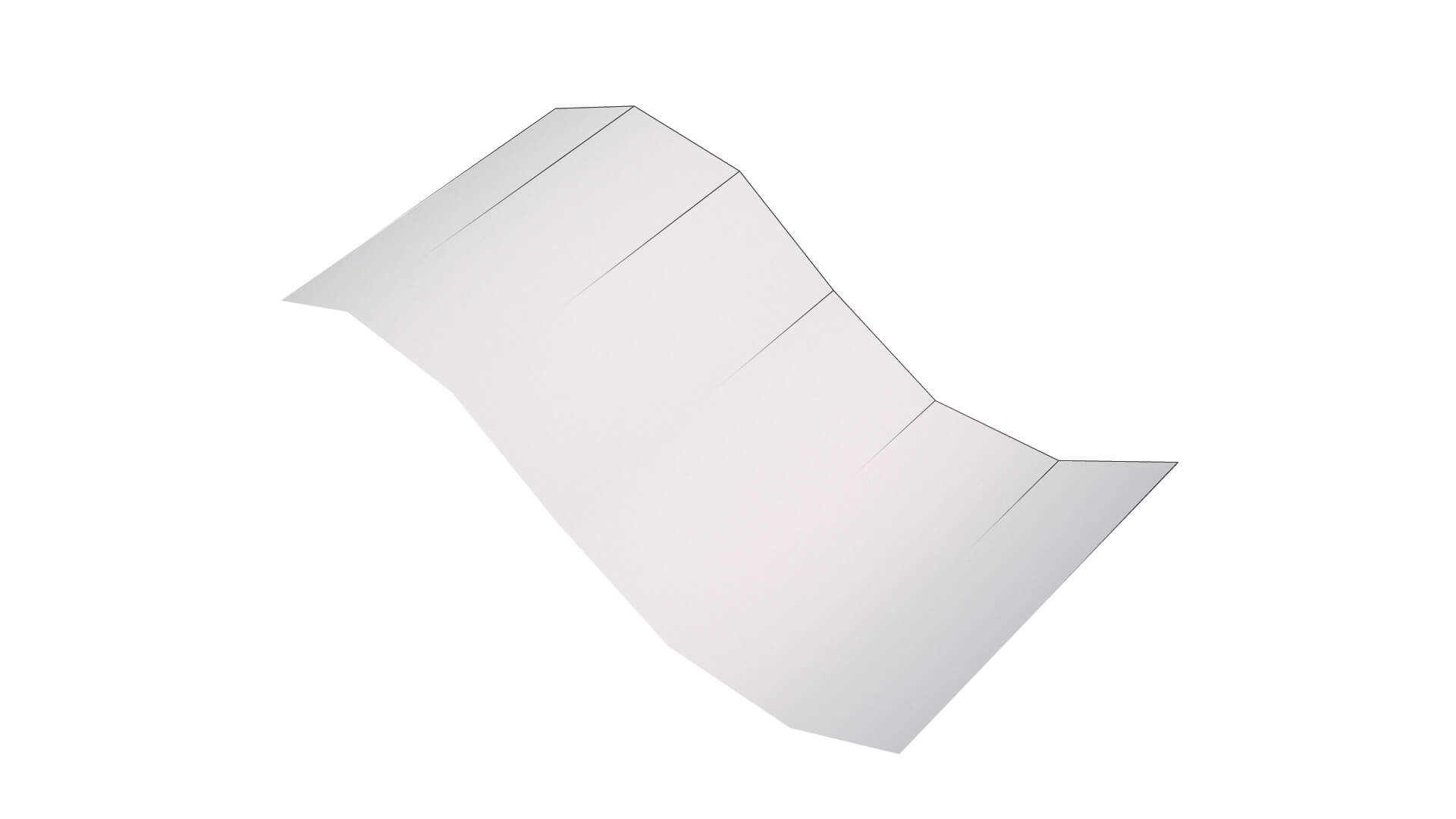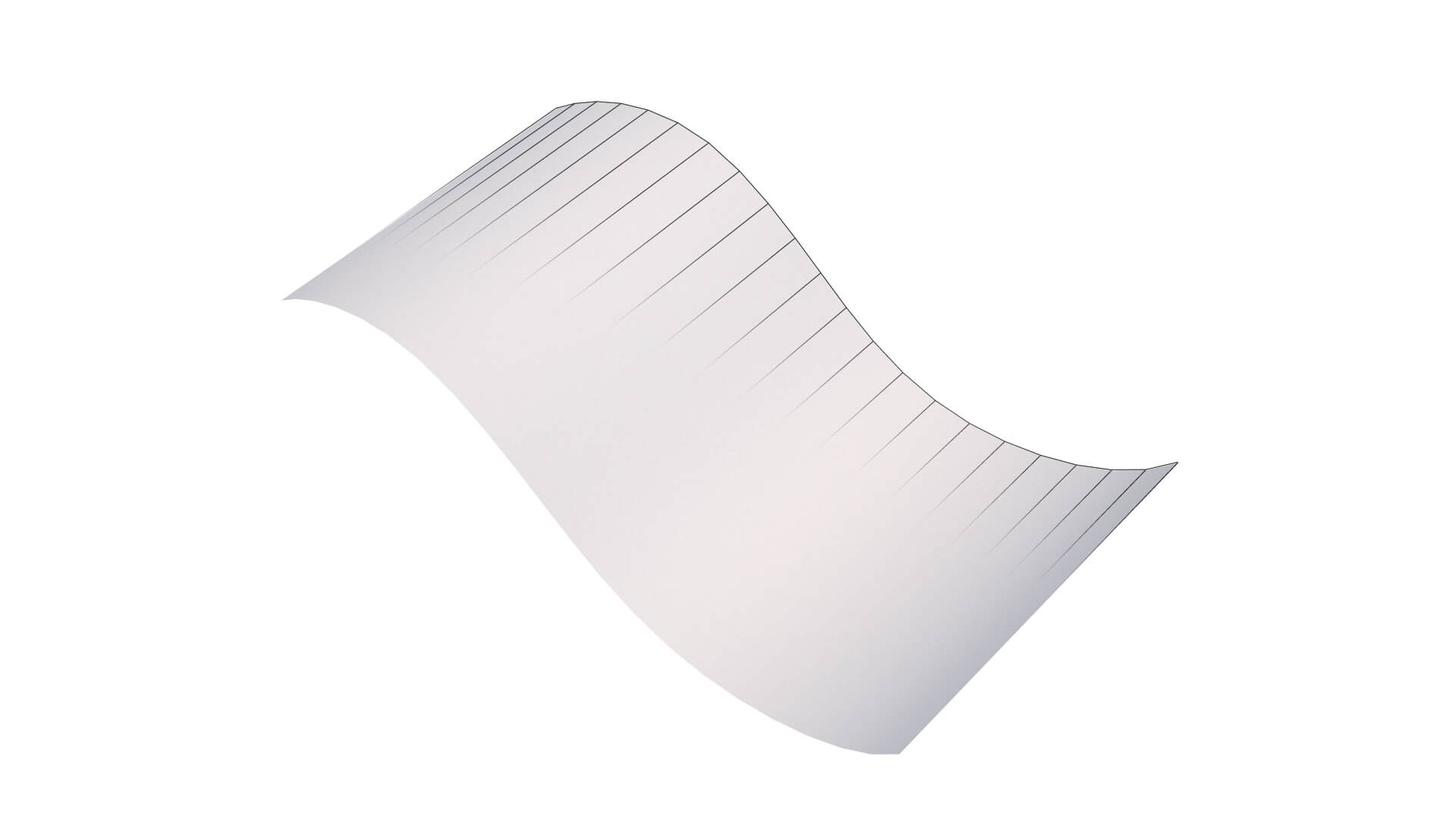# For the layman – How 3D Rendering Works. Part 1: 3D models

Welcome to this 1st part of what will most likely be a 7 part series on how 3D rendering works. It is an explanation for the layman. I will basically be touching upon all the elements of what is needed to construct a 3D render. 3D models, Materials, Textures, Camera & composition, Lighting, Rendering, and Post-processing. After each part I hope you will have gained a bit of insight what it is that is required to make a 3D render, and therefore what it is that me or any other 3D artist does to create the artwork that we make. I won’t be going in depth on any topic. This will probably be done in future articles. My main purpose is to spread some insight and awareness for those who are not familiar with 3D rendering. So let’s get started with this 1st part: 3D models.

For any 3D render that you make you will need a subject. For product visualizations this will be a 3D model of your product. Like in the thumbnail of this article. But what exactly is a 3D model? And are there different types of 3D models? These are questions I will be answering in this article.

### 1. What exactly is a 3D Model?

Well, what a 3D model basically is, is a computer generated mathematical representation of surfaces in a 3-Dimensional (3D) space that together form an object. In a computer program this 3D space is normally represented by a 3 axis orthogonal (the axis perpendicular to each other) coordinate system represented by an x-, y-, and z-axis. Like you can see in the image below of a 3D cup:Now there are 2 main methods to mathematically generate these surfaces. These are NURBS and Polygons. I will briefly explain both.

### 2. Polygon models

Most models are made up out of polygons. A polygon is nothing more than a surface build-up out of points in a 3d space. With edges connecting all the dots (like connect the dots when you were a kid). The closed of surface by the edges is then called a polygon. So let’s have a look at the example below.A cube is constructed out of 8 points. Each point we call a Vertex (Vertices for plural). There are a total of 12 lines that are connecting the vertices. These lines we call Edges. And the edges form a total of 6 square closed of surfaces. These surface we call Faces or Polygons. Each Face in this case is a square polygon. It has 4 vertices and 4 edges, and forms a square face. But a polygon can have more or less edges. As long as the edges make it a closed of surface. Like for example, in the image below you see that we can have squares, triangles or n-gons (which are whatever form polygon with a total of n-amount of edges).Now for professional work we normally work in square polygons to build up a model. This is because a lot of the computer tools we use to make 3D models can only really work when the model is build-up out of square polygons. When rendering in a 3D software package, models can often be converted during the rendering process into triangles.

So there you go. All the 3D artwork you see basically consists out of tiny little squares and triangles that form a larger complex object. Like the object below:### 3. NURBS (Non Uniform Rational B-Spline) models

The abbreviation of NURBS sounds a bit complex, but what NURBS basically are, are surfaces that are determined by mathematically defined curves in a 3D space. So as polygons are determined by points in a 3D space that are connected by edges to form polygon surfaces, NURBS are mathematically defined by curves in 3D space that form a surface when the curves are connected. This makes NURBS surfaces to be perfectly curved mathematical surfaces, compared to polygon surfaces which are basically stepwise edges/faces. Let’s look at the example NURBS surface below.We have 2 curves in 3D space. Each curve is determined by what you can consider a mathematical formula. Now if we were to connect the curves together, we obtain a mathematically determined surface in 3D space. This is a NURBS surface. Now if we were to have a third curve that represents how the surface should flow between the initial 2 curves, we can change how the NURBS surface is constructed, and thus how it looks between these initial 2 curves. This is what happens in the example below. This is basically what NURBS are. Mathematically defined surfaces defined by mathematically defined curves in a 3D space.
For example for the binoculars, all its separate parts can be built up by NURBS surfaces from curves that determine the surface shape of all the individual different parts.### 4. Tessellation

NURBS are mostly used in engineering and industrial software like CAM (Computer Aided Manufacturing), CAD (Computer Aided Design) and CAE (Computer Aided Engineering) software. The funny thing is, that when you make a 3D Render of NURBS surfaces, the NURBS surfaces are normally translated into polygon surfaces by the software, before the render starts. This process is called tessellation. Tessellation is also sometimes done when you import a NURBS model into a 3D render software. The higher the tessellation quality the better the polygon shape represents the original NURBS shape. But the higher the tessellation quality, the more polygons are used to represent the original NURBS shape, the heavier the model becomes and thus the longer rendering times become when you do your final 3D render. The rule is: More polygons equals longer render time. How rendering works will be explained in a subsequent blog post in this series. So for now you just have to take my word for it. Below you can see an example of the tessellation of a NURBS surface with high and low tessellation quality. As you can see, if the tessellation quality increases, the original NURBS surface is converted using more polygons and thus keeps its original shape better.### 5. 3D model formats

There are tons of different 3D modelling software out in the world. Each has its own file format. But there are a few general ones that I think you need to be aware of. These are the most common ones you will encounter. Or at least the most common ones I work with for myself and my clients:

• .OBJ (Object)- Most general 3D model format that contains only 3D polygon geometry data.
• .FBX (Filmbox)- Also a very general 3D polygon model format, but with the added benefit that it can contain data on basic materials (color and texture) defined on the object, and animation data.
• .STEP (STandard for the Exchange of Product data)- Most basic file format for CAD and CAM related models. This normally contains NURBS data from engineering software. These NURBS are tessellated into polygons when I import this model format in the 3D render software I use.
• So that is all of the basic knowledge you need to be aware of with regards to 3D models. Of course there is a lot more to know about 3D models, but this gives some basic insights and understanding of what it is that I work with to create all the amazing visuals for myself and my clients. I hope you have enjoyed this post and have learned something new. Let me know if you have any questions or comments below.